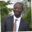Related Tags

communitycreator
php
sql
injection

# What is an SQL prepared statement in PHP?Abel Lifaefi Mbula

We have already discussed how to connect to an SQL database in PHP. In today’s shot, we want to see how to properly read from or write to the database.

### Example

Let’s consider this example. You have a basic form to collect user data like name, age, and country.

The code for the form is shown below.

<!DOCTYPE html>
<html lang="en">
<meta charset="UTF-8">
<meta http-equiv="X-UA-Compatible" content="IE=edge">
<meta name="viewport" content="width=device-width, initial-scale=1.0">
<title>User Info</title>
<body>
<h1>User Info</h1>
<form method="post">
<label for="name">Name</label>
<input type="text" placeholder="Your name here" name="name" id="name" required>

<label for="age">Age</label>
<input type="number" name="age" id="age" required>

<label for="country">Country</label>
<input type="text" placeholder="Your country here" name="country" id="country" required>

<input type="submit" name="submit" value="Send">
</form>
</body>
</html>
User info form

When a user submits this form, you may be tempted to do what is shown below in order to save data in the database.

$name =$_POST['name'];
$age =$_POST['age'];
$country =$_POST['country '];

$sql = "INSERT INTO users(name, age, country) VALUES($name, $age,$country);

// Query execution here


But code like this should not be put into a production app because it is vulnerable to SQL injection.

Note: You should never trust user inputs.

What’s the solution, then? We can use prepared statements with variable binding. Let’s see it in action.

### What you should do

We have to use the prepared statement also called the parameterized statement. A prepared statement is an SQL query template containing placeholder values instead of the actual parameter values.

Here’s how it works:

• Prepare the statement
• Execute it

Let’s look at it in detail.

#### Prepare the statement

At this step, a statement template is sent to the database server, which performs a syntax check and initializes server internal resources for later use.

To practice, we will use the prepare() method alongside with placeholders or markers. You can use either anonymous positional markers, such as ?, or named ones, such as (:name).

// anonymous markers
$stmt =$connection->prepare("INSERT INTO users(name, age, country) VALUES (?, ?, ?)");


You can use named markers, especially when you have many variables such as:

// named markers
$stmt =$connection->prepare("INSERT INTO users(name, age, country) VALUES (:name, :age, :country)");


#### Execute the statement

Once we have prepared our statement, the next step is to execute. Here, we bind parameter values and send them to the server which will execute the statement with the bound values using the previously created internal resources.

To bind parameters we use bind_param(type, $var): $stmt->bind_param("sis", $name,$age, $country); // "sis" means that$name is bound as a string, $age as an integer and$country as a string


s stands for string and corresponds to the first and the third parameters. i stands for integer and corresponds to the second parameter.

Note: Apart from s and i, you can also find d for double and b for blob.

We are now ready to execute our statement. To do so, we simply call execute() on our statement.

$stmt->execute();  ### Code Here’s the full code to handle the user submission as shown in the example at the beginning. <?php // make sure you have all database info$connection = new PDO($dsn,$username, $password,$options);

/* Step 1: prepare */
$sql = "INSERT INTO users(name, age, country) VALUES (?, ?, ?)"$stmt = $connection->prepare($sql);

/* Step 2: bind and execute */
$name =$_POST['name'];
$age =$_POST['age'];
$country =$_POST['country '];

$stmt->bind_param("sis",$name, $age,$country);

$stmt->execute(); Full code to handle a use submission ### How is a prepared statement safer? Let’s break down how SQL injection works and see how prepared statements are safer. The root of SQL injection problems comes from mixing code with data. Let’s say we want to select a student from the database by its id. $student_id = 1;
$sql = "SELECT * FROM students WHERE id=$student_id";


This will produce a regular query like this:

$sql = SELECT * FROM students WHERE id=1;  Sounds good, right? But what happens if the user or client sends spoiled data like this: $student_id = "1; DROP TABLE students;";
$sql = "SELECT * FROM students WHERE id=$student_id";


We will have a malicious query such as:

$sql = SELECT * FROM students WHERE id=1; DROP TABLE students;  As you can see here, we alter our program because we directly add data to its body. So, the protection we have with prepared statement is that we can separately send query and data to the database server. In short, we first send a program to the server without any data like this: $db->prepare("SELECT * FROM students WHERE id=?");


Then we can send data in the second request:

$db->execute($data);


In this way, data can’t alter our program or do any harm.

### Wrap it up

We’ve learned so far how you can write a code that is not vulnerable to SQL injection.

Here are the steps to follow:

• Send a statement template to the server without any (user) submitted data
$stmt =$connection->prepare("INSERT INTO users(name, age, country) VALUES (?, ?, ?)");

• Bind parameter values with data
$stmt->bind_param("sis",$name, $age,$country);

• Execute the statement
\$stmt->execute()


RELATED TAGS

communitycreator
php
sql
injection

CONTRIBUTORAbel Lifaefi Mbula
RELATED COURSES

View all Courses

Keep Exploring

Learn in-demand tech skills in half the time